Article citation information:

Dogan, E. Analysis and comparison of long short-term memory networks short-term traffic prediction performance. Scientific Journal of Silesian University of Technology. Series Transport. 2020, 107, 19-32. ISSN: 0209-3324. DOI: https://doi.org/10.20858/sjsutst.2020.107.2.

Erdem DOGAN

ANALYSIS AND COMPARISON OF LONG SHORT-TERM MEMORY NETWORKS SHORT-TERM TRAFFIC PREDICTION PERFORMANCE

Summary. Long short-term memory networks (LSTM) produces promising results in the prediction of traffic flows. However, LSTM needs large numbers of data to produce satisfactory results. Therefore, the effect of LSTM training set size on performance and optimum training set size for short-term traffic flow prediction problems were investigated in this study. To achieve this, the numbers of data in the training set was set between 480 and 2800, and the prediction performance of the LSTMs trained using these adjusted training sets was measured. In addition, LSTM prediction results were compared with nonlinear autoregressive neural networks (NAR) trained using the same training sets. Consequently, it was seen that the increase in LSTM's training cluster size increased performance to a certain point. However, after this point, the performance decreased. Three main results emerged in this study: First, the optimum training set size for LSTM significantly improves the prediction performance of the model. Second, LSTM makes short-term traffic forecasting better than NAR. Third, LSTM predictions fluctuate less than the NAR model following instant traffic flow changes.

Keywords: deep learning, traffic flow, short-term, prediction, LSTM, nonlinear autoregressive, training set size

1. INTRODUCTION

Nowadays, the number of vehicles and travel demands are increasing rapidly. This increase is responsible for delays, fuel loss and high emissions globally. For this reason, the efficiency of road capacities should be increased by directing and controlling road traffic with intelligent transport systems (ITS). However, ITS needs information about the current status of traffic variables and future estimates of this information (for example, volume, speed, travel time, etc.). For ITS to be more efficient, it is important that traffic parameters be accurately estimated, especially in the short term. Thus, ITS can make fast and accurate decisions for future traffic situations. For this reason, studies on predicting the short-term future situation of traffic become important. Researchers are working to make these predictions more accurate by developing new methods. Especially as deep learning has proven itself in many areas, the use of deep learning in short term traffic prediction has accelerated. Therefore, there is a need for research that better demonstrates the potentials of deep learning in this regard.

The first study on short-term traffic flow estimation was performed using the Box-Jenkins method . Time series methods were used to estimate traffic flow in other studies. [2-8] However, when artificial intelligence approaches and time series methods were compared, it was observed that artificial intelligence predicted short term traffic flow better . Therefore, in this study, traffic flow estimation models were developed by using artificial intelligence and deep networks approaches and the size of the training sets were discussed .

Short-term traffic flow estimation was performed with ANNs in earlier times from deep learning approach. For instance, the dynamic wavelet ANN model was used to estimate traffic flow . Dynamic traffic flow modelling is another approach to determine the amount of traffic flow . ANN and K-NN were used together to estimate traffic flow . In another study, multiscale analysis-based intelligent ensemble modelling was used to predict airway traffic . The traffic flow was modelled for the city of Istanbul using different time resolutions and the results were accurate despite the limited data  and some others [15-18]. Deep learning has recently gained interest in the prediction of various traffic parameters. Long short-term memory (LSTM) is in the sub-branch of deep learning. Previous studies on LSTM have evidence that deep learning and the performance of other methods were compared. For example, LSTM was compared with regression models . As a result, LSTM has generally made better predictions, except in some cases. Researchers developed a model using LSTM to predict the short-term traffic flow in exceptional traffic conditions. In addition, the authors studied the characteristics of traffic data . In another study, LSTM and recurrent ANN models were compared with ARIMA models . As a result, researchers mentioned that artificial intelligence models work better. In the other study, LSTM and recurrent ANN and regression models were compared with LSTM obtaining better results .

LSTM and short-term traffic flow were reviewed in the literature, but so far, there was no study on the effect of training set size on LSTM performance.  Therefore, in this study, LSTM and nonlinear autoregressive neural networks (NAR) were trained with different training sets size and the optimum size was determined for the problem. In addition, two models were compared, and their results discussed. Thus, the results of this study will help to determine the size of the training set for future studies.

This article consists of introduction, methodology and conclusion. The subject and importance of this study are discussed, and the related literature is summarised in the introduction section of this article. The data used and the estimation of missing data are presented in the methodology section. Then, LSTM and NAR approaches were briefly explained, and the parameters of the models used in the study were introduced. Thereafter, NAR and LSTM estimates were tested by hypothesis testing and the results were discussed. Finally, the conclusions of the study were recalled in the conclusion section and recommendations were made for future studies.

1. METHODOLOGY

1.1.       Data collection and missing data

Traffic flow data were collected from the D200 highway. This highway connects the major cities of Turkey. The main road traffic is not interrupted 20 km forward and backward from the counted section. Therefore, there are uninterrupted conditions in the counting section. Data collection was performed with NC-350 traffic counters . The counting was conducted with traffic counting devices placed separately for the left and right lanes. The devices were set to record data every 15 minutes. Devices were counted for 47 days and 4,512 traffic flow data were collected.

In the counting process, data cannot be recorded at some time intervals and this is very common. This data is called missing data. This is often the result of faults in the device or the limitations of the counting device. After counting operations, it was found that approximately 1% of the total data was missing. Autocorrelation reveals the degree of relationship of time series points with each other. The points with high autocorrelation are used in making future predictions. To complete the missing data, traffic data with high autocorrelation were used with missing data. The results of the autocorrelation calculation result are given in Fig. 1. Autocorrelation was high at point 672. Each counting operation has 15 min intervals. In other words, every point in the time series is related to the point 7 days (672 / (24 * 4) previous. This is a very common pattern in traffic flows.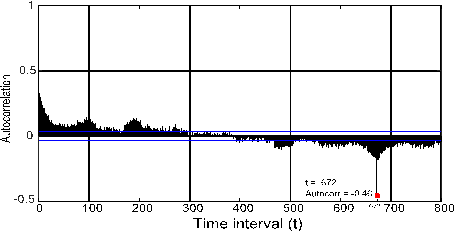Fig. 1. Completion of missing data

In this case, the missing data can be completed with the value at the point 672 interval before the missing point. Let X be traffic data with missing values and xt X indicates the traffic flow data at time t. Also, let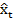denote the missing data in the series and at time t. According to these definitions, the missing data is completed as in Equation 1.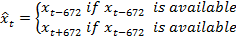(1)

After completing the missing data, the data set was standardised with Equation 2 before training the models.(2)

where,

xstd       standardised data,

x          raw data,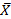mean of the dataset,

sx         standard deviation of the dataset.

1.2.            Long short-term memory

Long short-term memory network (LSTM) is an advanced type of recurrent neural networks (RNNs) that can overcome the long-term dependence problem. RNNs produced successful results in sequence prediction tasks. However, it is often difficult for RNNs to learn long-term patterns . LSTM can understand short- or long-term dependencies with the help of units that learn when to forget and when to update the information.

Let xt be the input vector, ht be the output of the LSTM unit and Ct be the cell state at time t.  In the first step, how much of the information in the Ct-1 will be forgotten is determined by forget gate. The forget gate is a layer that uses sigmoid function and uses ht-1 and xt to generate values between “0” and “1”. Therefore, ft in Fig. 2 can be written as: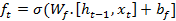(3)

The next step is to identify new information that will be stored in the cell state. This step consists of two sub-steps: The first step is the input gate, which determines what information to update. The second step determines the vector containing the candidate values. In Fig. 2, the output value of the input gate is represented by it, while the output value of the second section is indicated by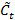. The it andcan be written as:and